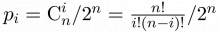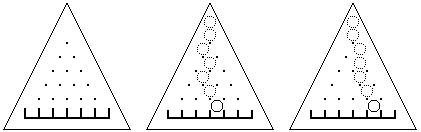## Day 1, Problem 2 - Nails & Ball

On a triangular wooden board placed upright, there are n(n + 1)/2 nails, and (n + 1) slots (the first figure below depicts the board for n = 5). The distance from each nail to a surrounding nail is d, and the width of each slot is also d. With the exception of the leftmost and rightmost slots, each slot perfectly aligns with the spaces formed by the bottommost row of nails.

Let a ball of radius d start at the center of the topmost nail of the board and naturally roll down. Every time the ball hits a nail, it has the chance of falling towards the left or towards the right (each has a 1/2 probability), directly on top of the next nail below it. The second figure below depicts one possible route for the ball.

We know that the probability of the ball landing in the i-th slot is$\textstyle p_{i}=\textrm{C}_{n}^{i}/2^{n}=\frac{n!}{i!(n-i)!}/2^{n}$, where the slots are numbered 0, 1, …, n from left to right.

After pulling out some nails, the problem now is to find the probability pm of the ball landing in slot m. Assume that no nails will be pulled from the bottommost row. The third figure below depicts one possible way that the ball can fall after some nails have been removed.### Input Format

The first line of input contains the two integers n (2 ≤ n ≤ 50) and m (0 ≤ mn). The remaining n lines depict the n rows of nails on the board. A '*' indicates that the nail is still there, while a '.' indicates that the nail has been pulled out. Note that among each of these n lines, spaces may appear anywhere.

### Output Format

Output one line containing a reduced fraction (0 is written as 0/1), the probability pm of the ball landing in slot m. A reduced fraction is defined as the value A/B, where A and B are integers that do not share any common factor greater than 1.

### Sample Input

5 2
*
* .
* * *
* . * *
* * * * *


### Sample Output

7/16


Point Value: 15 (partial)
Time Limit: 1.00s
Memory Limit: 16M